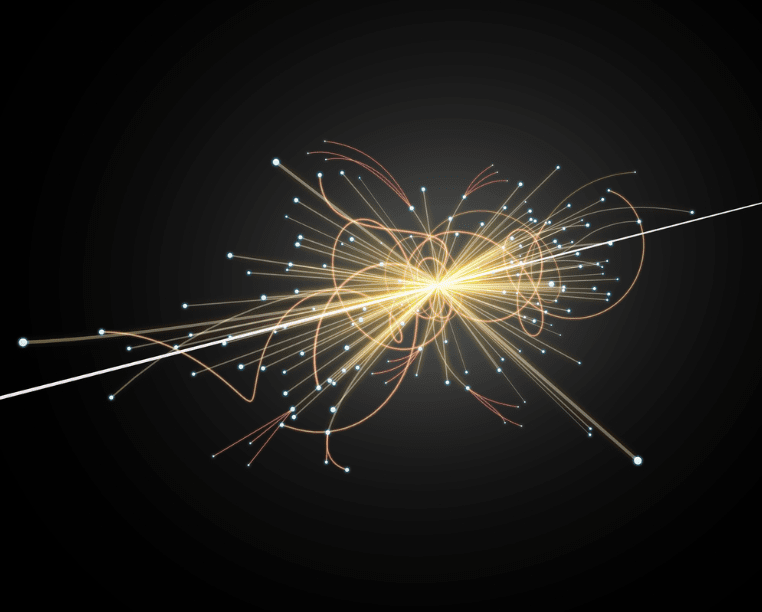Coffee Room
Discuss anything here - everything that you wish to discuss with fellow engineers.
12763 Members
Join this group to post and comment.Does "E=mc2" theory rules out in the case of "LARGE HYDROGEN COLLIDER" process?when the particles gets collided in "LHC"
Energy released during that collision does equate with "E=mc2" theory (or) "E=mc2" rules out!!!Shashank Moghe • Mar 31, 2016when particles in "LHC" collide a lot of energy(E) is released.Now in E=mc2 on substituting values for E,m,c does it satisfies or rules out !!!Shashank Moghe • Apr 4, 2016
E=mc2 gives the maximum energy potential of a rest mass "m". This is the limit of the energy you can get from this mass. When particles collide and energy is released, a fraction of the mass is converted into energy. Similar conversion occurs in many chemical and nuclear reactions. If you are asking whether Conservation of Energy is violated, it is not.thats the real generalization
but how does collision of a small or negligible mass of those particles would equate to enormous energy(E) released in E=mc2Shashank Moghe • Apr 5, 2016
c = 299792458 m/s (source: Google)
c^2 = 89875517873681764 m^2/s^2

Multiply by what you might call a "negligible" mass with this, lets say a milligram (10^-6 kg).

E = 10^-6 * c^2 = 89875517873.681764 Joules

Not small, is it?the calculation was perfect!
but the mass of particle means the mass of proton(mp)
mp=1.6726219*10^-27 kg that matters.

now the calculated value moves on to
E=[1.6726219*10^-27]*
which is very smallShashank Moghe • Apr 6, 2016
The experiments at LHC are designed to accelerate sub-atomic particles to relativistic speeds (near that of c), a process that requires an extremely huge amount of energy. You might ask why does it need so much energy? As you approach c, the relativistic mass approaches infinity. Hence, the force required to accelerate a particle to c (no matter how less massive, even a proton!) will be infinite! We can only accelerate the particles in the LHC (or any other particle accelerator) to a value close to c (but never c), and even that requires massive amounts of energy. That is why such experiments are called extremely high energy experiments. This energy gets translated into the kinetic energy of the particle.

The formula (E=mc2) gives only the energy potential based on the rest mass of that photon. It does not say anything about its energy content when it is in motion (keep in mind the word "rest mass" used in that equation). Imagine the photon being accelerated in the LHC to near c values. Its mass will be huge and its kinetic energy will be even larger! And when two such particles collide? I rest my case.

This link gives a much better description that I ever can:
https://www.einstein-online.info/elementary/specialRT/emcokay!!!
i got the point of explanation.
thank you and a lot of threads are strugling in my mind to get a correct explanation...